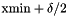#Boost C++ Libraries

...one of the most highly regarded and expertly designed C++ library projects in the world.

## Class template discrete_distribution

boost::random::discrete_distribution

## Synopsis

```// In header: <boost/random/discrete_distribution.hpp>

template<typename IntType = int, typename WeightType = double>
class discrete_distribution {
public:
// types
typedef WeightType input_type;
typedef IntType    result_type;

// member classes/structs/unions

class param_type {
public:
// types
typedef discrete_distribution distribution_type;

// construct/copy/destruct
param_type();
template<typename Iter> param_type(Iter, Iter);
param_type(const std::initializer_list< WeightType > &);
template<typename Range> explicit param_type(const Range &);
template<typename Func> param_type(std::size_t, double, double, Func);

// public member functions
std::vector< WeightType > probabilities() const;

// friend functions
template<typename CharT, typename Traits>
friend std::basic_ostream< CharT, Traits > &
operator<<(std::basic_ostream< CharT, Traits > &, const param_type &);
template<typename CharT, typename Traits>
friend std::basic_istream< CharT, Traits > &
operator>>(std::basic_istream< CharT, Traits > &, const param_type &);
friend bool operator==(const param_type &, const param_type &);
friend bool operator!=(const param_type &, const param_type &);
};

// construct/copy/destruct
discrete_distribution();
template<typename Iter> discrete_distribution(Iter, Iter);
discrete_distribution(std::initializer_list< WeightType >);
template<typename Range> explicit discrete_distribution(const Range &);
template<typename Func>
discrete_distribution(std::size_t, double, double, Func);
explicit discrete_distribution(const param_type &);

// public member functions
template<typename URNG> IntType operator()(URNG &) const;
template<typename URNG> IntType operator()(URNG &, const param_type &) const;
result_type min() const;
result_type max() const;
std::vector< WeightType > probabilities() const;
param_type param() const;
void param(const param_type &);
void reset();

// friend functions
template<typename CharT, typename Traits>
friend std::basic_ostream< CharT, Traits > &
operator<<(std::basic_ostream< CharT, Traits > &,
const discrete_distribution &);
template<typename CharT, typename Traits>
friend std::basic_istream< CharT, Traits > &
operator>>(std::basic_istream< CharT, Traits > &,
const discrete_distribution &);
friend bool operator==(const discrete_distribution &,
const discrete_distribution &);
friend bool operator!=(const discrete_distribution &,
const discrete_distribution &);
};```

## Description

The class `discrete_distribution` models a random distribution . It produces integers in the range [0, n) with the probability of producing each value is specified by the parameters of the distribution.

### `discrete_distribution` public construct/copy/destruct

1. `discrete_distribution();`

Creates a new `discrete_distribution` object that hasand.

2. `template<typename Iter> discrete_distribution(Iter first, Iter last);`

Constructs a `discrete_distribution` from an iterator range. If `first` == `last`, equivalent to the default constructor. Otherwise, the values of the range represent weights for the possible values of the distribution.

3. `discrete_distribution(std::initializer_list< WeightType > wl);`

Constructs a `discrete_distribution` from a `std::initializer_list`. If the `initializer_list` is empty, equivalent to the default constructor. Otherwise, the values of the `initializer_list` represent weights for the possible values of the distribution. For example, given the distribution

``` discrete_distribution<> dist{1, 4, 5};
```

The probability of a 0 is 1/10, the probability of a 1 is 2/5, the probability of a 2 is 1/2, and no other values are possible.

4. `template<typename Range> explicit discrete_distribution(const Range & range);`

Constructs a `discrete_distribution` from a Boost.Range range. If the range is empty, equivalent to the default constructor. Otherwise, the values of the range represent weights for the possible values of the distribution.

5. ```template<typename Func>
discrete_distribution(std::size_t nw, double xmin, double xmax, Func fw);```

Constructs a `discrete_distribution` that approximates a function. If nw is zero, equivalent to the default constructor. Otherwise, the range of the distribution is [0, nw), and the weights are found by calling fw with values evenly distributed betweenand, where.

6. `explicit discrete_distribution(const param_type & param);`

Constructs a `discrete_distribution` from its parameters.

### `discrete_distribution` public member functions

1. `template<typename URNG> IntType operator()(URNG & urng) const;`

Returns a value distributed according to the parameters of the `discrete_distribution`.

2. ```template<typename URNG>
IntType operator()(URNG & urng, const param_type & param) const;```

Returns a value distributed according to the parameters specified by param.

3. `result_type min() const;`

Returns the smallest value that the distribution can produce.

4. `result_type max() const;`

Returns the largest value that the distribution can produce.

5. `std::vector< WeightType > probabilities() const;`

Returns a vector containing the probabilities of each value of the distribution. For example, given

``` discrete_distribution<> dist = { 1, 4, 5 };
std::vector<double> p = dist.param();
```

the vector, p will contain {0.1, 0.4, 0.5}.

6. `param_type param() const;`

Returns the parameters of the distribution.

7. `void param(const param_type & param);`

Sets the parameters of the distribution.

8. `void reset();`

Effects: Subsequent uses of the distribution do not depend on values produced by any engine prior to invoking reset.

### `discrete_distribution` friend functions

1. ```template<typename CharT, typename Traits>
friend std::basic_ostream< CharT, Traits > &
operator<<(std::basic_ostream< CharT, Traits > & os,
const discrete_distribution & dd);```

Writes a distribution to a `std::ostream`.

2. ```template<typename CharT, typename Traits>
friend std::basic_istream< CharT, Traits > &
operator>>(std::basic_istream< CharT, Traits > & is,
const discrete_distribution & dd);```

Reads a distribution from a `std::istream`

3. ```friend bool operator==(const discrete_distribution & lhs,
const discrete_distribution & rhs);```

Returns true if the two distributions will return the same sequence of values, when passed equal generators.

4. ```friend bool operator!=(const discrete_distribution & lhs,
const discrete_distribution & rhs);```

Returns true if the two distributions may return different sequences of values, when passed equal generators.